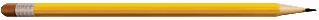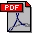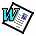## PUBLICATIONS By Professor CherkasskySelecting the loss function for robust linear regression (ABSTRACT)Submitted to Neural Computation, 2002 Multiple Model Estimation: A New Formulation for Predictive Learning (ABSTRACT)Submitted to IEEE Transaction on Neural Networks, 2002 SVM-based Learning for Multiple Model Estimation> (ABSTRACT)Submitted to IEEE Transaction on Neural Networks, 2002 Model Complexity Control and Statistical Learning Theory (ABSTRACT)Natural Computing: An International Journal, Kluwer, 1,1, 109-133, 2002 Comparison of Model Selection for Regression (ABSTRACT)Submitted to Neural Computation, 2002 Practical Selection of SVM Parameters and Noise Estimation for SVM Regression (ABSTRACT)Submitted to Neurocomputing, Special Issue on SVM, 2002 Rigorous Application of VC Generalization Bounds to Signal Denoising (ABSTRACT)Submitted to IEEE Transactions on Neural Networks, 2002### Selecting the loss function for robust linear regression

#### Abstract

This paper addresses selection of the loss function for regression problems with finite data. It is well-known (under standard regression formulation) that for a known noise density there exist an optimal loss function under an asymptotic setting (large number of samples), i.e. squared loss is optimal for Gaussian noise density. However, in real-life applications the noise density is unknown and the number of training samples is finite. Robust statistics provides prescriptions for choosing the loss function using only general information about noise density; however robust statistics is based on asymptotic arguments and may not work well for finite sample problems. For such practical situations, we suggest using Vapnik's -insensitive loss function. We propose a practical method for setting the value of as a function of known number of samples and (known or estimated) noise variance. First we consider commonly used unimodal noise densities (such as Gaussian and Laplacian). Empirical comparisons for several representative linear regression problems indicate that the proposed loss function yields more robust performance and improved prediction accuracy, in comparison with commonly used squared loss and least-modulus loss, especially for noisy high-dimensional data sets. We also performed comparisons for symmetric bimodal noise densities (where large errors occur more likely than small errors). For such (bimodal) noise, the proposed -insensitive loss consistently provides improved prediction accuracy (in comparison with other loss functions), for both low-dimensional and high-dimensional problems.

### Multiple Model Estimation: A New Formulation for Predictive Learning

#### Abstract

This paper presents a new formulation for predictive learning called multiple model estimation. Existing learning methodologies are based on traditional formulations such as classification or regression, which assume that available (training) data is generated by a single (unknown) model. The deviations from this model are treated as zero-mean i.i.d. noise. These assumptions about underlying statistical model for data generation are somewhat relaxed in robust statistics, where a small number of outliers is allowed in the training data. However, the goal of learning (under robust statistical formulations) remains the same, i.e., estimating a single model consistent with the majority of 'representative' training data. In many real-life applications it is natural to assume multiple models underlying an unknown system under investigation. In such cases, training data is generated by different (unknown) statistical models. Hence, the goal of learning is to simultaneously solve two problems, i.e. to estimate several statistical models AND to partition available (training) data into several subsets (one subset for each underlying model). This paper presents generic mathematical formulation for multiple model estimation. We also discuss several application settings where proposed multiple model estimation is more appropriate than traditional single-model formulations.

### Multiple Model Estimation: A New Formulation for Predictive Learning

#### Abstract

This paper presents new constructive learning methodology for multiple model estimation. Under multiple model formulation, training data are generated by several (unknown) statistical models, so existing learning methods (for classification or regression) based on a single model formulation are no longer applicable. We describe general framework for multiple model estimation using SVM methodology. The proposed constructive methodology is analyzed in detail for regression formulation. We also present several empirical examples for multiple-model regression formulation. These empirical results illustrate advantages of the proposed multiple model estimation approach.

### Model Complexity Control and Statistical Learning Thoery

#### Abstract

We discuss the problem of model complexity control also known as model selection. This problem frequently arises in the context of predictive learning and adaptive estimation of dependencies from finite data. First we review the problem of predictive learning as it relates to model complexity control. Then we discuss several issues important for practical implementation of complexity control, using the framework provided by Statistical Learning Theory (or Vapnik-Chervonenkis theory). Finally, we show practical applications of Vapnik-Chervonenkis (VC) generalization bounds for model complexity control. Empirical comparisons of different methods for complexity control suggest practical advantages of using VC-based model selection in settings where VC generalization bounds can be rigorously applied. We also argue that VC-theory provides methodological framework for complexity control even when its technical results can not be directly applied.

### Comparison of Model Selection for Regression

#### Abstract

We discuss empirical comparison of analytical methods for model selection. Even though there exist plenty of analytic model selection criteria, there is no consensus on which method works best for practical (finite-sample) estimation problems, even for the simple case of linear estimators. This paper presents empirical comparisons between classical statistical methods (AIC, BIC) and the SRM method (based on VC-theory) for regression problems. Our study is motivated by empirical comparisons in [Hastie et al, 2001] who claim that SRM method performs poorly for model selection. Hence, we present empirical comparisons for different data sets and different types of estimators (linear, subset selection and k-nearest neighbor regression). Our results demonstrate practical advantages of VC-based model selection, as it consistently outperforms AIC and BIC for most data sets (including those used in [Hastie et al, 2001]). This discrepancy (between empirical results obtained using the same data) appears to be caused by methodological drawbacks in [Hastie et al, 2001]) especially in their loose interpretation and application of the SRM method. Hence we discuss methodological issues important for meaningful comparisons and practical application of SRM method.

### Practical Selection of SVM Parameters and Noise Estimation for SVM Regression

#### Abstract

We investigate practical selection of meta-parameters for SVM regression (that is, -insensitive zone and regularization parameter C). The proposed methodology advocates analytic parameter selection directly from the training data, rather than resampling approaches commonly used in SVM applications. Good generalization performance of the proposed parameter selection is demonstrated empirically using several low-dimensional and high-dimensional regression problems. Further, we point out the importance of Vapnik's -insensitive loss for regression problems with finite samples. To this end, we compare generalization performance of SVM regression (with optimally chosen ) with regression using 'least-modulus' loss ( =0). These comparisons indicate superior generalization performance of SVM regression, for finite sample settings.

### Rigorous Application of VC Generalization Bounds to Signal Denoising

#### Abstract

Recently, several empirical studies showed practical application of VC-bounds for regression for model selection with linear estimators. In this paper we discuss issues related to practical model complexity control using VC-bounds for nonlinear estimators, i.e. minimization of the empirical risk and accurate estimation of the VC-dimension. Then we present an application setting (signal denoising) where the empirical risk can be reliably minimized. However, with adaptive signal denoising (aka wavelet thresholding) an accurate estimation of the VC-dimension becomes difficult. For such signal denoising applications, we propose practical modification of VC-bounds for model selection. Effectively, the proposed approach provides a heuristic methodology for estimating the VC-dimension as a function of the number of orthogonal basis functions (wavelet or Fourier) used for signal representation. Then this VC-dimension can be used in VC-analytic bounds for model selection, for determining an optimal number of orthogonal basis functions for a given (noisy) signal. The proposed (heuristic) methodology called improved VC signal denoising provides better estimation accuracy than the original VC-denoising approach and other popular thresholding methods for representative univariate signals.